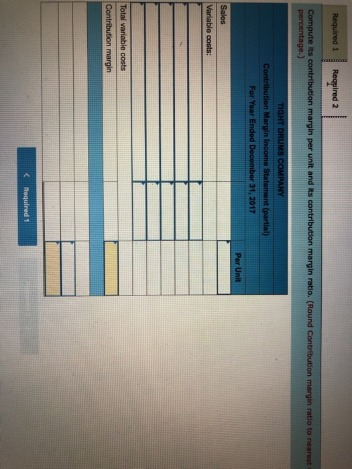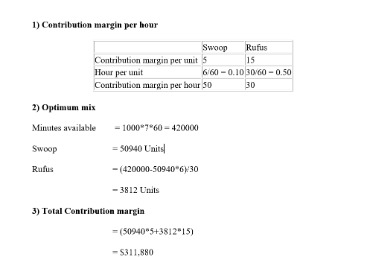# How to calculate contribution per unitFor this section of the exercise, the key takeaway is that the CM requires finding the revenue from the sale of a specific product line, along with the specific variable costs. While there are various profitability metrics – ranging from the gross margin down to the net profit margin – the contribution margin (CM) metric stands out for the analysis of a specific product/service. The Contribution Margin represents the revenue from a product minus direct variable costs, which results in the incremental profit earned on each unit of product sold. The contribution margin is a managerial ratio that is used to determine the breakeven point for a product and from there they can make informed decisions on product pricing. A business can also use this ratio to close down product lines that are not showing profits and invest in profitable product lines instead.

Just as with any other financial ratio, the calculation of the contribution margin could yield a low or higher value. When a business registers a higher contribution margin value, it would mean that it has enough resources available to meet its variable as well as fixed costs. 1) It helps you determine how much money your business generates on every dollar of sales. You can use this information to determine whether your business is profitable or not and whether it is growing or not (if your contribution margin percentage changes). When a firm decides which products to offer or which markets to penetrate, it should examine each product’s contribution margins to determine if it will contribute enough profit to cover its fixed costs.

## What is the difference between contribution margin and contribution margin ratio?

Put simply, when a business manufactures a product or offers a given service, there is a cost attached to it. When you subtract that variable cost of delivering a product from the sales price of the product, the remainder is the contribution margin. Use the contribution margin to help you establish the monthly break-even point before you become profitable. The break-even point is the minimum number of units you must sell to account for production costs and all other fixed costs.

Contribution per unit is the residual profit left on the sale of one unit, after all variable expenses have been subtracted from the related revenue. This information is useful for determining the minimum possible price at which to sell a product. In essence, never go below a contribution per unit of zero; you would otherwise lose money with every sale. The only conceivable reason for selling at a price that generates a negative contribution margin is to deny a sale to a competitor. The contribution margin measures the difference between the sales price of a product and the variable costs per unit.

## What is a contribution margin ratio?

To calculate the formula for the unit contribution margin expressed as a dollar value, use revenues per unit subtracted by variable expenses per unit. To express this as a percentage ratio, take the resulting number and divide it by the revenues per unit. Calculating the unit contribution margin uses the total revenues, minus the variable costs divided by the total number of units. You can use this calculator to calculate the contribution margin for a company by entering the value of net sales and variable costs. The second element of the contribution margin formula is the variable costs. Variable costs are costs that change in a company with an increase in production.The difference between the two is that the contribution margin ratio is the percentage of sales revenue that goes towards covering the variable costs. While the contribution margin is the total dollar value of the money left over after variable costs have been deducted. Enter the selling price per unit, variable cost per unit, and the total number of units sold into the contribution margin calculator.

## How to calculate contribution per unit

This tool is essential in helping to determine how much money is available for distribution to owners as dividends and how much money is available for reinvestment in the company. This means that if you sell 10 products, your total contribution would be \$250. If your cost of goods sold was also \$250, then you would achieve 100% contribution per sales ratio on that item.

• Use the contribution margin to help you establish the monthly break-even point before you become profitable.
• Our work has been directly cited by organizations including MarketWatch, Bloomberg, Axios, TechCrunch, Forbes, NerdWallet, GreenBiz, Reuters, and many others.
• Through the calculation of the variable costs and profit per unit sold, they can use the analysis to predict the estimates for the upcoming year.

The 60% ratio means that the contribution margin for each dollar of revenue generated is \$0.60. Instead of looking at the profitability of a company on an overall basis with all products grouped together, the CM enables margin analysis on an individual product line basis. The actual calculation of contribution margin may be more laborious but the concept applies.

## Contribution per Unit Calculator

Variable costs are not typically shown on company financial statements and the usual way to get the figures is to tally them up from the income statement. It’s not common to issue income statements that split variable and fixed costs, but some companies do separate them. Examples of variable costs include the cost of raw materials, cost of labour, shipping etc. The more the business produces the more it will spend on raw materials and labour, and that is the reason why this cost varies with an increase in production. Contributions margin ratio (also known as gross profit ratio) is one of the most important financial ratios.

3) You can use contribution margins for setting prices for different services offered by your business. This will help you establish fair prices that are attractive for patients and cover the cost of providing care. To go through a simple example, let’s say there’s an e-commerce company selling t-shirts for \$25.00 with variable costs of \$10.00 per unit. The calculation of the metric is relatively straightforward, as the formula consists of revenue minus variable costs. For companies seeking to obtain a sustainable long-term competitive advantage, it’s important to focus on identifying the products with the highest contribution margins in order to maximize potential profits.